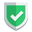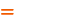我们相信：世界是美好的，你是我也是。平行空间的世界里面，不同版本的生活也在继续...

`react`项目，也可能会使用到`setInverval`类型代码，这类代码的功用是每隔几秒不间断执行一段代码。如果在这代码体内执行更新`state`的操作，却发生了意想不到的事情，特殊情况下，会得到`state`更新失败的结果。那么，如何解决这种问题呢？这就是本文要描述的话题。

## 测试代码

``````import { useState, useRef } from "react";
function Child() {
const [count, setCount] = useState(0);
let timerRef = useRef(null);
if (timerRef.current === null) {
timerRef.current = setInterval(() => {
setCount((count + 1) % 6);
}, 1000);
}
const sunan_do = () => {
setCount((count + 1) % 6);
};
return (
<div className="Child">
函数式组件测试,{count},
<button onClick={sunan_do.bind()}>手工加1</button>
</div>
);
}
export default Child;``````

``setCount((count + 1) % 6);``

## 解决方案

``setCount((count + 1) % 6);``

``setCount((count) => (count + 1) % 6);``

``````setCount((count) => {
let count2 = (count + 1) % 6;
return count2;
});``````

## 类式组件中不存在这种问题

``````import React, { Component } from 'react'
export default class ChildCompare extends Component {
constructor(props) {
super(props);
this.state = { count: 0 };
this.timerRef = React.createRef(null);
}
render() {
if (this.timerRef.current == null) {
this.timerRef.current = setInterval(() => {
let count2 = (this.state.count + 1) % 9;
// this.setState("count", count2);
this.setState({ count: count2 });
}, 1000);
}
return (
<div>类式组件测试,{this.state.count}</div>
)
}
}``````

## 结束语如果本文对您有帮助，或者节约了您的时间，欢迎打赏瓶饮料，建立下友谊关系。本博客不欢迎：各种镜像采集行为。请尊重原创文章内容，转载请保留作者链接。【福利】 腾讯云最新爆款活动！1核2G云服务器首年50元！【源码】本文代码片段及相关软件，请点此获取更多信息【绝密】秘籍文章入口，仅传授于有缘之人react